Generating function of product of bivariate Hermite polynomials and their applications in studying quantum optical states*
Department of Physics, Ningbo University, Ningbo 315211, China
Department of Material Science and Engineering, University of Science and Technology of China, Hefei 230026, China
Department of Modern Physics, University of Science and Technology of China, Hefei 230026, China
College of Science, Changzhou Institute of Technology, Changzhou 213002, China

Corresponding author. E-mail: fhym@ustc.edu.cn

*Project supported by the National Natural Science Foundation of China (Grant No. 11175113), the Fundamental Research Funds for the Central Universities of China (Grant No. WK2060140013), and the Natural Science Foundation of Jiangsu Higher Education Institution of China (Grant No. 14KJD140001).

Abstract

By virtue of the operator-Hermite-polynomial method, we derive some new generating function formulas of the product of two bivariate Hermite polynomials. Their applications in studying quantum optical states are presented.

Keyword: 03.65.–w; 42.50.–p; 03.67.–a; operator-Hermite-polynomials (OHP) method; generating function; product of bivariate Hermite polynomials
1. Introduction

In quantum mechanics and mathematical physics, the special function, the Hermite polynomial, is of fundamental importance. Physically, it is the eigenfunction of the harmonic oscillator. In addition, by introducing a convenient complex form of the α -th two-dimensional fractional Fourier transform operation, it is found that the bivariate Hermite polynomial is a new eigenmode that propagates in quadratic graded index media. Mathematically, the single-variable Hermite polynomial Hn(x) can be defined in terms of its generating function,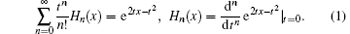In Ref. , by combining the operator-Hermite-polynomials (OHP) method and the technique of integration within an ordered product (IWOP) of operators,  we derived the generating functions of even and odd Hermite polynomials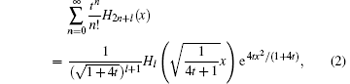when l = 0, 1. Since H0(x) = 1, H1(x) = 2x, we have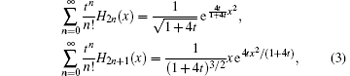and these formulas are not only accumulated in mathematical handbooks but can also be useful in constructing some optical quantum states. Furthermore, by introducing the Hermite-polynomial-operator Hn(X), where X is the coordinate operator (or the quadrature operator in quantum optics theory), and combining the IWOP technique, some new operator identities about quantum squeezing are derived, which are useful for studying the squeezed number state. On the other hand, by replacing the arguments of the special function by quantum mechanical operators, a binomial theorem involving Hermite polynomials and a negative-binomial theorem involving Laguerre polynomials have been derived. These two theorems will have essential applications in quantum optics calculations, and the method is concise and helpful in deducing many operator identities, which may become a new branch in mathematical physics theory. Using the entangled state representation, a two-mode squeezed number state can be converted into a Hermite polynomial excited squeezed vacuum state. The generalized photon-added coherent state is obtained by repeatedly acting the combination of Bose creation and annihilation operations on the coherent state. It is found that it can be regarded as a Hermite excited coherent state due to its normalization factor related to Hermite polynomials.

For the bivariate Hermite polynomials Hm, n(x, y) (note that Hm, n is not a direct product of two independent single-variable Hermite polynomials)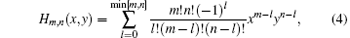with the generating function in the form of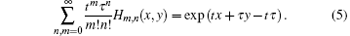The bivariate Hermite polynomials Hm, n have their own applications in studying quantum optics. For example, in reference to the Weyl ordering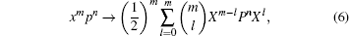where X and P are coordinate and momentum operator, respectively. In Ref. , we examined the operators’ parameterized ordering and its classical correspondence, and found the fundamental function-operator correspondence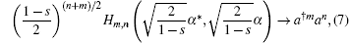and its complementary relation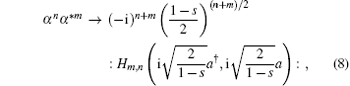where the symbol : : denotes normal ordering.

In Ref.  we derived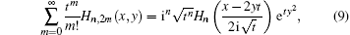and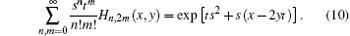These two equations are the generating functions of Hn, 2m (x, y) and the more complicated one is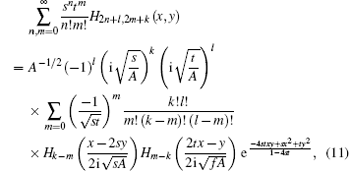where A = 1 – 4st.

When k = l = 0, we have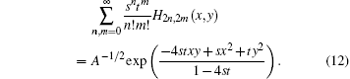In this work we shall derive some new generating function formula of the product of bivariate Hermite polynomials (PBHP), and we shall employ the OHP method to realize our goal. By the OHP method in this work, we aim to replace the classical Hermite polynomials Hm, n(x, y) by the operator ⋮ Hm, n(a, a)⋮ first, where a and a are the Bose creation and annihilation operator, respectively, obeying [a, a] = 1, ⋮ ⋮ denotes anti-normal ordering. By using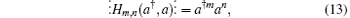we can derive some operator identities in definite operator ordering (proof is given in Appendix A). Then we convert them back to the classical case. We also present some applications of these new generating function formulas of PBHP in quantum optics. Next, we shall briefly review the OHP method and exhibit its convenience and simplicity.

2. Brief review of the OHP method

One of the fundamental operator identities in the OHP method is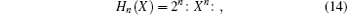where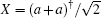. We can use it to search for the generating function of the product Hn(x)Hn(y); namely, we replace Hn(x) by Hn(X). By using Eqs. (1) and (14), then we have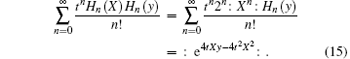Again by using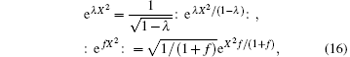we have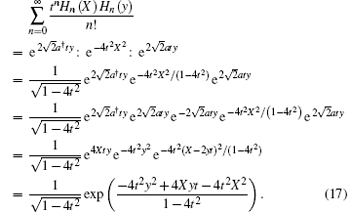Converting X back to x, we obtain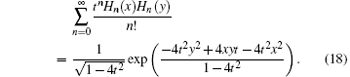Thus, the merit of using the OHP method is fully displayed.

3. One-index-summation of the product of bivariate Hermite polynomials

Now we prove the following one-index-summation of the product of two bivariate Hermite polynomials: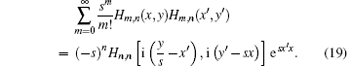In this case, the OHP method we use is to replace Hm, n(x′ , y′ ) by ⋮ Hm, n(a, a)⋮ .

Proof Rewrite Eq. (5) as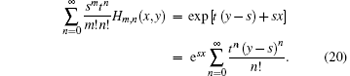By comparing the same power term of tn/n! on the two sides we conclude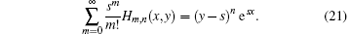Then we employ the OHP method to consider the summation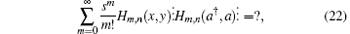where ⋮ Hm, n(a, a)⋮ is in the anti-normal ordering form. Noting that a and a are permuted within the anti-normal ordering symbol ⋮ ⋮ and using Eqs. (13) and (21) we can re-express Eq. (22) as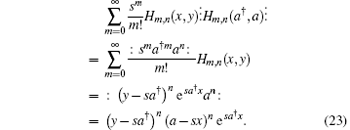Then we need to change the right-hand side of Eq. (23) into anti-normal ordering for comparing with the left-hand side. Using the identity which is responsible for converting an operator ρ (a, a) into its anti-normally ordered form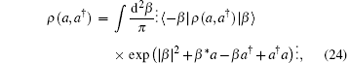where | β 〉 = exp(− | β | 2/2 + β a) | 0〉 is a coherent state, 〈 − | β 〉 = e– 2| β | 2, we try to put (ysa)n (asx)n into its anti-normal ordering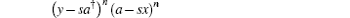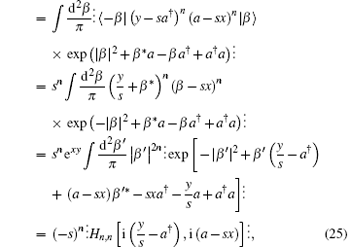where in the last step we have used the integration formula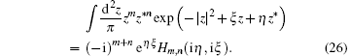Substituting Eq. (25) into Eq. (23) we obtain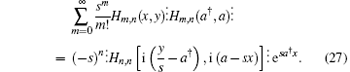Since both sides in Eq. (18) are in anti-normal ordering and a, a are commutable within ⋮ ⋮ , we can replace ax′ , ay′ , leading to Eq. (19).

Based on the relation to the Laguerre polynomials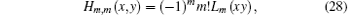where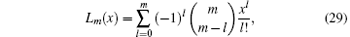we can obtainSimilarly, we can prove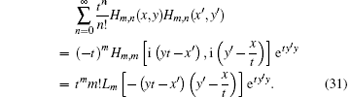4. Summation formula of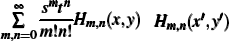By using the generating function of Laguerre polynomials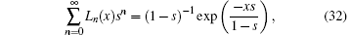we can further make summation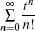for Eq. (30) and obtain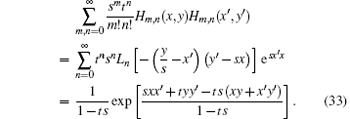This formula can be used to derive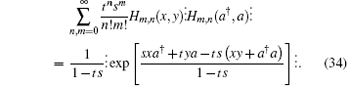On the other hand, using Eq. (13) it is equal to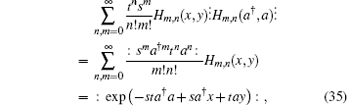we obtain a new operator identity which links normal ordering to anti-normal ordering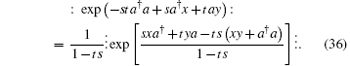In particular, when x = y = 0,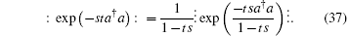5. Generating function of Hm+ l, n+ k(x, y)

Next we examine the summation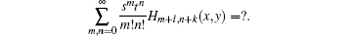To proceed, using the OHP method and Eq. (13) we instead consider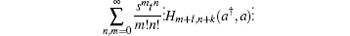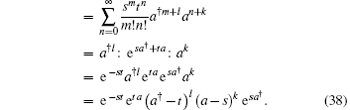Using the binomial theorem regarding the bivariate Hemite polynomials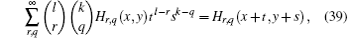we have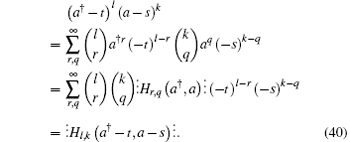Thus, equation (29) becomesSince the both sides are in anti-normal ordering, we have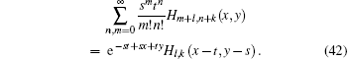This is another type of generating function of bivariate Hermite polynomials.

6. Applications in analyzing quantum optical states

As an application of Eq. (30), we consider the two-mode photon-subtracted squeezed vacuum state ambmS2(λ )| 00〉 , where S2(λ ) = exp [λ (abab)] is the two-mode squeezing operator, with λ being a real squeezing parameter. The two-mode squeezed state is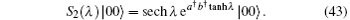Using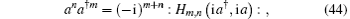its derivation is shown in Appendix A. From Eq. (30), we obtain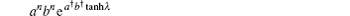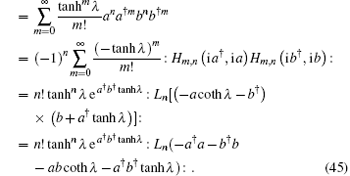Thus, the two-mode photon-subtracted squeezed vacuum state can be expressed as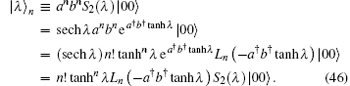Therefore, | λ n is equivalent to Laguerre polynomial excitation on the two-mode squeezed vacuum state. In Ref. , we calculated its normalization factor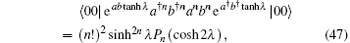where Pm(x) is the Legendre polynomial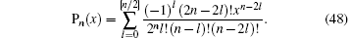Using Eqs. (46) and (47) as well as the coherent state’ s completeness relation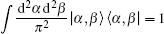we have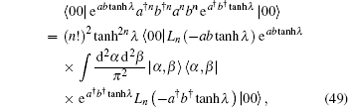sowhich is a new integration formula.

As an application of Eq. (33), we obtain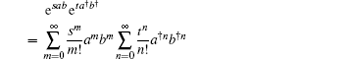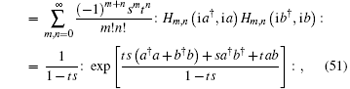which changes the anti-normally ordered form esab etab into its normal ordering, or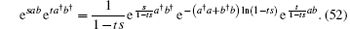Then we turn to an application of Eq. (42) in quantum optics. We consider the following state vector which is generated by operating Hl, k (ξ b, ξ * – a) on the entangled state | ξ 〉 , where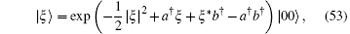which takes the bivariate Hermite polynomials Hm, n(ξ , ξ * ) as its expansion function in the two-mode Fock space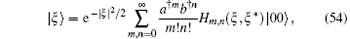| ξ 〉 obeys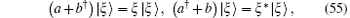so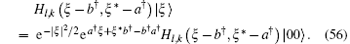In comparison with Eq. (42) we see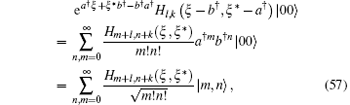where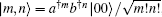, so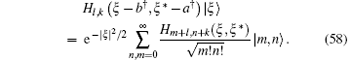It follows the Fock space wave function of Hl, k (ξ b, ξ * – a) | ξ 〉 that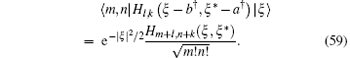7. Conclusion

In summary, by virtue of the OHP method and the IWOP technique, we have derived some new generating function formulas about the bivariate Hermite polynomials. Their applications in studying quantum optics are presented as well.

Reference
 1 Lu H L and Fan H Y 2007 Commun. Theor. Phys. 47 1024 DOI:10.1088/0253-6102/47/6/011 [Cited within:1] 2 Fan H Y and Fan Y 2003 Commun. Theor. Phys. 39 97 DOI:10.1088/0253-6102/39/1/97 [Cited within:1] 3 Fan H Y and Zhan D H 2014 Chin. Phys. B 23 060301 DOI:10.1088/1674-1056/23/6/060301 [Cited within:2] [JCR: 1.148] [CJCR: 1.2429] 4 Fan H Y, Lou S Y, Pan X Y and Da C 2014 Acta Phys. Sin. 63 110304 DOI:10.7498/aps.63.110304(in Chinese) [Cited within:1] [JCR: 1.016] [CJCR: 1.691] 5 Fan H Y and Lou S Y 2013 Sci. China-Phys. Mech. Astron. 56 2042 DOI:10.1007/s11433-013-5311-2 [Cited within:1] 6 Fan H Y, Zhan D H, Yu W J and Zhou J 2012 Acta Phys. Sin. 61 110302(in Chinese) http://118.145.16.217/magsci/article/article?id=17394526 [Cited within:1] [JCR: 1.016] [CJCR: 1.691] 7 Fan H Y, Lou S Y, Pan X Y and Da C 2012 Acta Phys. Sin. 61 110302(in Chinese) http://118.145.16.217/magsci/article/article?id=17394526 [Cited within:1] [JCR: 1.016] [CJCR: 1.691] 8 Hu L Y, Wang S and Zhang Z M 2012 Chin. Phys. B 21 064207 DOI:10.1088/1674-1056/21/6/064207 [Cited within:1] [JCR: 1.148] [CJCR: 1.2429] 9 Li H M and Xu X F 2012 Chin. Phys. B 21 024202 DOI:10.1088/1674-1056/21/2/024202 [Cited within:1] [JCR: 1.148] [CJCR: 1.2429] 10 Fan H Y, He R, Da C and Liang Z F 2013 Chin. Phys. B 22 080301 DOI:10.1088/1674-1056/22/8/080301 [Cited within:1] [JCR: 1.148] [CJCR: 1.2429] 11 Fan H Y, Yuan H C and Hu L Y 2010 Chin. Phys. B 19 104204 DOI:10.1088/1674-1056/19/10/104204 [Cited within:1] 12 Zhan D H Fan H Y Chin. Phys. B in press [Cited within:1] 13 Fan H Y 2012 Sci. China-Phys. Mech. Astron. 55 762 DOI:10.1007/s11433-012-4699-4 [Cited within:1] 14 Fan H Y 1991 Phys. Lett. A 161 1 [Cited within:1] [JCR: 1.11] 15 Loudon R and Knight P L 1987 J. Mod. Opt. 34 709 DOI:10.1080/09500348714550721 [Cited within:2] 16 Zhang Z X and Fan H Y 1992 Phys. Lett. A 165 14 DOI:10.1016/0375-9601(92)91046-T [Cited within:1] [JCR: 1.11] 17 Fan H Y and Klauder J R 1988 J. Phys. A 21 L725 DOI:10.1088/0305-4470/21/14/003 [Cited within:1] [JCR: 2.771] 18 Fan H Y and Fan Y 2001 Phys. Lett. A 282 269 DOI:10.1016/S0375-9601(01)00198-0 [Cited within:1] [JCR: 1.11]